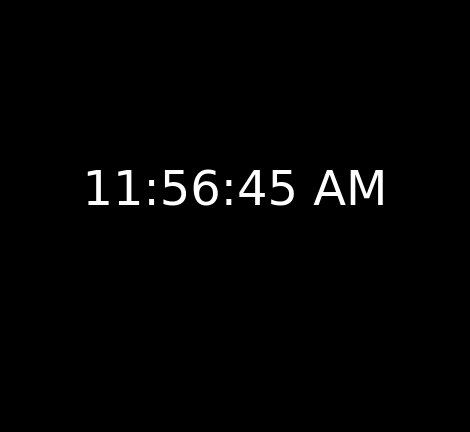Open in App
Not now

# How to make a digital clock in p5.js ?

• Difficulty Level : Medium
• Last Updated : 08 Mar, 2021

The working of the clock is simple. The current hours, minutes and seconds is retrieved using the hour(), minute() and second() methods. The number returned is then padded with a “0” if it is less than 10 using the formatting function we will create. The AM or PM is determined by using the detected hours value. The whole time string is then displayed as a text using the text() method on the canvas.

Example:

## Javascript

 `function` `setup() {`` ` `  ``// Create Canvas of given size``  ``createCanvas(480,480);``}`` ` `function` `draw() {`` ` `  ``// Set the background color``  ``background(0);``   ` `  ``// Set the strokeWeight to be thicker``  ``strokeWeight(4);``   ` `  ``// Get the current second, minute and hours``  ``// and assign them to res variables``  ``var` `sec = second();``  ``var` `min = minute();``  ``var` `hrs = hour();``   ` `  ``// Check for AM or PM based on the``  ``// hours and store it in a variable``  ``var` `mer = hrs < 12 ? ``"AM"``:``"PM"``;``   ` `  ``// Format the time so that leading``  ``// 0's are added when needed``  ``sec = formatting(sec);``  ``min = formatting(min);``  ``hrs = formatting(hrs % 12);``   ` `  ``// Set the color of the background ``  ``fill(255);``   ` `  ``// Set the font size ``  ``textSize(48);``   ` `  ``// Set the text alignment in center``  ``// and display the result``  ``textAlign(CENTER, CENTER);``   ` `  ``// Display the time``  ``text(hrs + ``":"` `+ min + ``":"` `+ sec +``       ``" "` `+ mer, width/2, height/2);``}`` ` `// This function pads the time``// so that 0's are shown ``// eg. 06,08,05 etc.``function` `formatting(num){``   ` `  ``// Convert to int and check ``  ``// if less than 10``  ``if``(int(num) < 10) {``     ` `    ``// Return the padded number``    ``return` `"0"` `+ num;``  ``}``   ` `  ``// Return the original number if``  ``// padding is not required``  ``return` `num;``}`

Output:Online Code editor:https://editor.p5js.org/

My Personal Notes arrow_drop_up